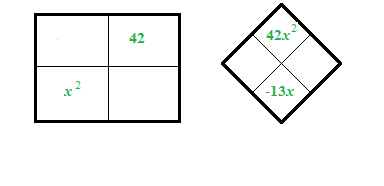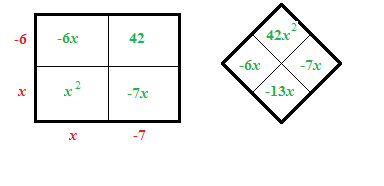### Home > INT2 > Chapter 5 > Lesson 5.2.4 > Problem5-100

5-100.

1. $x^2 − 13x + 42 = 0$

Draw an area model and diamond. Generic rectangle labeled as follows: Interior upper right box is negative 42. Interior lower left box is X squared. Diamond problem: Left blank, Right blank, Top 42, x squared, Bottom negative 13, x.

Factor. Added to the rectangle: Interiors: upper left, negative 6, x, & lower right, negative 7, x. Exteriors: left edge, top, negative 6, & bottom, x, bottom edge, left, x, & right, negative 7. Added to the diamond problem: Left negative 6, x, Right negative 7, x.

$(x − 6)(x − 7) = 0$

$x = 6$.
Make sure you find the other solution.1. $0 = 3x^2 + 10x - 8$

$0 = (3x − 2)(?\: + \:?)$

1. $2x^2 − 10x = 0$

There are only two terms, so factor using the GCF.

1. $4x^2 + 8x − 60 = 0$

Notice that each term is a multiple of $4$.

$x = 3$ or $x = −5$